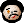# 2023-01-23 TODO stats table with parameters

Last time I wrote about my TODO stats table I promised to make it more configurable. And here I am.

Let’s start with deciding what parameters I want. Looking at the section about clock tables in the Org mode manual, I can see three parameters I could use here, too:

• `:maxlevel`
• `:scope`
• `:match`

Here is how you can implement them. Probably the easiest one is `:maxlevel` – you can call `(org-reduced-level (org-current-level))` on a headline to get its level. (Here, “reduced” means “taking into account the fact that someone might use `org-odd-levels-only`.) Note that `org-current-level` returns nil when the point is before the first headline, so I’ll take that into account, too.

It is also not difficult to implement `:scope` – it is, after all, one of the parameters of the `org-map-entries` function. Beware, though – the possible scopes for e.g. the clock table and that function are slightly different! The first difference is that clock table’s `:scope` allows a function which would return the list of files. The second, more important difference is that in the clock table’s `:scope`, `subtree` means “current subtree”, `treeN` means “the surrounding level N tree” and `tree` means `tree1`. In the case of `org-map-entries`​’ scope parameter, `tree` means “the current subtree” and there are no `subtree` nor `treeN` options. So, in order to define a clock-table-like `:scope` parameter, we need to pass it to `org-map-entries` unless it is one of `subtree`, `tree`, `treeN`, or a function. In order to do that, we can copy some code from the `org-dblock-write:clocktable` function.

The situation is the easiest with `:match` – you can simply pass it to `org-map-entries`, and that’s it. The only improvement I can envision here is that I could create another, Boolean parameter to turn on property inheritance just for the one stats table being constructed. This could be achieved by adding the (special) variable `org-use-property-inheritance` to the `let` clause surrounding the call to `org-map-entries`. I leave it as an exercise for the reader.

So, let’s put all this knowledge into practice (and code). A bit surprisingly, I couldn’t find an Elisp function to go up the Org file structure until a certain level, so I wrote one. The code should be pretty self-explanatory. And now I have a way to see the status of all my TODO items in a neat table. Even better, this is now a part of the dynamic block system, so e.g. `C-u C-c C-x C-u` automatically recomputes all such tables along the clock tables. (Now that I learned how to code a custom dynamic block, I am thinking about other possibilities like this…)

```(defun org-up-to-level (&optional level)
"Go up to a headline at LEVEL (default 1)."
(interactive "p")
(setq level (or level 1))
(while (> (org-reduced-level (or (org-current-level) 0))
level)

(defun org-subtree-todo-stats (maxlevel match scope)
MAXLEVEL, MATCH and SCOPE are like in clock table."
(save-excursion
(let ((stats (mapcar (lambda (keyword) (cons keyword 0))
org-todo-keywords-1))
(ome-scope (cond
((eq scope 'subtree) 'tree)
((eq scope 'tree) (progn (org-up-to-level) 'tree))
((string-match "\\`tree\\([0-9]+\\)\\'"
(symbol-name scope))
(org-up-to-level
(string-to-number
(match-string 1
(symbol-name scope))))
'tree)
((functionp scope) (funcall scope))
(t scope))))
(org-map-entries
(lambda ()
(when (or (null maxlevel)
(<= (org-reduced-level (org-current-level))
maxlevel))
(let ((keyword (org-element-property
:todo-keyword
(org-element-at-point))))
(when keyword
(cl-incf (alist-get keyword stats nil nil #'string=))))))
match
ome-scope 'archive 'comment)
stats)))

(defun org-dblock-write:todotable (params)
"Write a todotable."
(insert "| Keyword | Count |\n")
(insert "|-\n")
(mapc (lambda (keyword-count)
(insert (format
"| %s | %s |\n"
(car keyword-count)
(cdr keyword-count))))
(org-subtree-todo-stats (plist-get params :maxlevel)
(plist-get params :match)
(plist-get params :scope)))
(org-table-align))
```